# Difference between revisions of "Sinc function"

This article is about a particular function from a subset of the real numbers to the real numbers. Information about the function, including its domain, range, and key data relating to graphing, differentiation, and integration, is presented in the article.
View a complete list of particular functions on this wiki
For functions involving angles (trigonometric functions, inverse trigonometric functions, etc.) we follow the convention that all angles are measured in radians. Thus, for instance, the angle of$90\,^\circ$ is measured as$\pi/2$.

## Definition

This function, denoted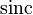$\operatorname{sinc}$, is defined as follows:$\operatorname{sinc} x := \left\lbrace \begin{array}{rl} 1, & x = 0 \\ \frac{\sin x}{x} & x \ne 0\end{array}\right.$

## Key data

Item Value
default domain all real numbers, i.e., all of$\R$.
range the closed interval$[\alpha,1]$ where$\alpha$ is approximately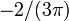$-2/(3\pi)$.
period none; the function is not periodic
local maximum values and points of attainment The local maxima occur at points$x$ satisfying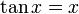$\tan x = x$ and$x in [2n\pi,(2n + 1)\pi]$ or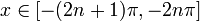$x \in [-(2n + 1)\pi,-2n\pi]$ for$n$ a positive integer.
There is an anomalous local maximum at$x = 0$ with value 1. Apart from that, the other local maxima occur at points of the form$\pm(2n\pi + \alpha_n)$ where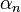$\alpha_n$ is fairly close to$\pi/2$ for all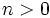$n > 0$. The local maximum value at this point is slightly more than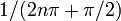$1/(2n\pi + \pi/2)$.
local minimum values and points of attainment The local minima occur at points$x$ satisfying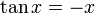$\tan x = -x$ and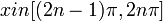$x in [(2n - 1)\pi,2n\pi]$ or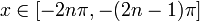$x \in [-2n\pi,-(2n - 1)\pi]$ for$n$ a positive integer.
The local minima occur at points of the form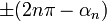$\pm(2n\pi - \alpha_n)$ where$\alpha_n$ is fairly close to$\pi/2$ for all$n > 0$. The local minimum value at this point is slightly less than$-1/(2n\pi - \pi/2)$.
points of inflection Fill this in later
derivative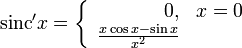$\operatorname{sinc}'x = \left\lbrace \begin{array}{rl} 0, & x = 0 \\ \frac{x \cos x - \sin x}{x^2} \\\end{array}\right.$

## Graph

Below is a graph of the function for the domain restricted to$[-3\pi,3\pi]$:

The picture is a little unclear, so we consider an alternative depiction of the graph where the$x$-axis and$y$-axis are scaled differently to make it clearer:

## Graph

Below is a graph of the function for the domain restricted to$[-3\pi,3\pi]$:

The picture is a little unclear, so we consider an alternative depiction of the graph where the$x$-axis and$y$-axis are scaled differently to make it clearer: